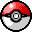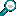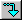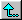Caitlyn's Pokémon Card Collection

 Cards  -->Find Card by -->  Name  |  Move  |  Type  | Characters  -->Find Character by -->  Name  |

All Pokémon Characters

Display options
 Sort by: Evolution Stage TypeNameRegionRecord ID Display: Comments   Record ID

page  of 81 to 100 of 728

A  B  C  D  E  F  G  H  I  J  K  L  M  N  O  P  Q  R  S  T  U  V  W  X  Y  Z

 AAbomasnow
Evolution Stage = Stage 1   Region = Sinnoh   Type = Grass, Ice   Size = 7'3", 298.7 lbs   Caitlyn's rating = 3 (good)

Abra
Evolution Stage = Basic   Region = Kanto   Type = Psychic   Size = 2'11", 43 lbs   Caitlyn's rating = 2 (fair)

Evolution Stage = Basic   Region = Hoenn   Type = Darkness   Size = 3'11", 103.6 lbs   Caitlyn's rating = 5 (favorite)

Accelgor
Evolution Stage = Stage 1   Region = Unova   Type = Bug   Size = 2'7", 55.8 lbs

Aegislash
Evolution Stage = Stage 2   Region = Kalos   Type = Metal, Ghost   Size = 5'7", 116.8 lbs

Aerodactyl
Evolution Stage = Basic   Region = Kanto   Type = Rock, Flying   Size = 5'11", 130.1 lbs   Caitlyn's rating = 3 (good)

Aggron
Evolution Stage = Stage 2   Region = Hoenn   Type = Metal, Rock   Size = 6'11", 793.7 lbs   Caitlyn's rating = 3 (good)

Aipom
Evolution Stage = Basic   Region = Johto   Type = Colorless   Size = 2'7", 25.4 lbs   Caitlyn's rating = 3 (good)

Alakazam
Evolution Stage = Stage 2   Region = Kanto   Type = Psychic   Size = 4'11", 105.8 lbs   Caitlyn's rating = 4 (excellent)

Alomomola
Evolution Stage = Basic   Region = Unova   Type = Water   Size = 3'11", 69.7 lbs

Altaria
Evolution Stage = Stage 1   Region = Hoenn   Type = Dragon, Flying   Size = 3'7", 45.4 lbs   Caitlyn's rating = 5 (favorite)

Amaura
Evolution Stage = Basic   Region = Kalos   Type = Rock, Ice   Size = 4'3", 55.6 lbs

Ambipom
Evolution Stage = Stage 1   Region = Sinnoh   Type = Colorless   Size = 3'11", 44.8 lbs   Caitlyn's rating = 3 (good)

Amoonguss
Evolution Stage = Stage 1   Region = Unova   Type = Grass, Poison   Size = 2', 23.1 lbs

Ampharos
Evolution Stage = Stage 2   Region = Johto   Type = Lightning   Size = 4'7", 135.6 lbs   Caitlyn's rating = 4 (excellent)

Anorith
Evolution Stage = Basic   Region = Hoenn   Type = Rock, Bug   Size = 2'4", 27.6 lbs   Caitlyn's rating = 2 (fair)

Arbok
Evolution Stage = Stage 1   Region = Kanto   Type = Poison   Size = 11'6", 143.3 lbs   Caitlyn's rating = 4 (excellent)

Arcanine
Evolution Stage = Stage 1   Region = Kanto   Type = Fire   Size = 6'3", 341.7 lbs   Caitlyn's rating = 4 (excellent)

Evolution Stage = Basic   Region = Sinnoh   Type = Colorless   Size = 10'6", 705.5 lbs   Caitlyn's rating = 4 (excellent)

Archen
Evolution Stage = Basic   Region = Unova   Type = Rock, Flying   Size = 2', 20.9 lbs

Archeops
Evolution Stage = Stage 1   Region = Unova   Type = Rock, Flying   Size = 4'8", 70.5 lbs

Evolution Stage = Stage 1   Region = Johto   Type = Bug, Poison   Size = 3'7", 73.9 lbs   Caitlyn's rating = 4 (excellent)

Armaldo
Evolution Stage = Stage 1   Region = Hoenn   Type = Rock, Bug   Size = 4'11", 150.4 lbs   Caitlyn's rating = 3 (good)

Aromatisse
Evolution Stage = Stage 1   Region = Kalos   Type = Fairy   Size = 2'7", 34.2 lbs

Aron
Evolution Stage = Basic   Region = Hoenn   Type = Metal, Rock   Size = 1'4", 132.3 lbs   Caitlyn's rating = 2 (fair)

Evolution Stage = Basic   Region = Kanto   Type = Ice, Flying   Size = 5'7", 122.1 lbs   Caitlyn's rating = 4 (excellent)

Audino
Evolution Stage = Basic   Region = Unova   Type = Colorless   Size = 3'7", 68.3 lbs

Aurorus
Evolution Stage = Stage 1   Region = Kalos   Type = Rock, Ice   Size = 8'10", 496 lbs

Avalugg
Evolution Stage = Stage 1   Region = Kalos   Type = Ice   Size = 6'7", 1113.3 lbs

Axew
Evolution Stage = Basic   Region = Unova   Type = Dragon   Size = 2', 39.7 lbs

Azelf
Evolution Stage = Basic   Region = Sinnoh   Type = Psychic   Size = 1', 0.7 lbs   Caitlyn's rating = 3 (good)

Azumarill
Evolution Stage = Stage 1   Region = Johto   Type = Water   Size = 2'7", 62.8 lbs   Caitlyn's rating = 5 (favorite)

Azurill
Evolution Stage = Baby   Region = Hoenn   Type = Colorless   Size = 8", 4.4 lbs   Caitlyn's rating = 3 (good)

 BBagon
Evolution Stage = Basic   Region = Hoenn   Type = Dragon   Size = 2', 92.8 lbs   Caitlyn's rating = 3 (good)

Baltoy
Evolution Stage = Basic   Region = Hoenn   Type = Ground, Psychic   Size = 1'8", 47.4 lbs   Caitlyn's rating = 2 (fair)

Banette
Evolution Stage = Stage 1   Region = Hoenn   Type = Ghost   Size = 3'7", 27.6 lbs   Caitlyn's rating = 3 (good)

Barbaracle
Evolution Stage = Stage 1   Region = Kalos   Type = Rock, Water   Size = 4'3", 211.6 lbs

Barboach
Evolution Stage = Basic   Region = Hoenn   Type = Water, Ground   Size = 1'4", 4.2 lbs   Caitlyn's rating = 2 (fair)

Basculin
Evolution Stage = Basic   Region = Unova   Type = Water   Size = 3'3", 39.7 lbs

Bastiodon
Evolution Stage = Stage 1   Region = Sinnoh   Type = Rock, Metal   Size = 4'3", 329.6 lbs   Caitlyn's rating = 3 (good)

Bayleef
Evolution Stage = Stage 1   Region = Johto   Type = Grass   Size = 3'11", 34.8 lbs   Caitlyn's rating = 4 (excellent)

Beartic
Evolution Stage = Stage 1   Region = Unova   Type = Ice   Size = 8'6", 573.2 lbs

Beautifly
Evolution Stage = Stage 2   Region = Hoenn   Type = Bug, Flying   Size = 3'3", 62.6 lbs   Caitlyn's rating = 3 (good)

Beedrill
Evolution Stage = Stage 2   Region = Kanto   Type = Bug, Poison   Size = 3'3", 65 lbs   Caitlyn's rating = 3 (good)

Beheeyem
Evolution Stage = Stage 1   Region = Unova   Type = Psychic   Size = 3'3", 76.1 lbs

Beldum
Evolution Stage = Basic   Region = Hoenn   Type = Metal, Psychic   Size = 2', 209.9 lbs   Caitlyn's rating = 2 (fair)

Bellossom
Evolution Stage = Stage 2   Region = Johto   Type = Grass   Size = 1'4", 12.8 lbs   Caitlyn's rating = 3 (good)

Bellsprout
Evolution Stage = Basic   Region = Kanto   Type = Grass, Poison   Size = 2'4", 8.8 lbs   Caitlyn's rating = 2 (fair)

Bergmite
Evolution Stage = Basic   Region = Kalos   Type = Ice   Size = 3'3", 219.4 lbs

Bibarel
Evolution Stage = Stage 1   Region = Sinnoh   Type = Colorless, Water   Size = 3'3", 69.4 lbs   Caitlyn's rating = 3 (good)

Bidoof
Evolution Stage = Basic   Region = Sinnoh   Type = Colorless   Size = 1'8", 44.1 lbs   Caitlyn's rating = 3 (good)

Binacle
Evolution Stage = Basic   Region = Kalos   Type = Rock, Water   Size = 1'8", 68.3 lbs

Bisharp
Evolution Stage = Stage 1   Region = Unova   Type = Darkness, Metal   Size = 5'3", 154.3 lbs

Blastoise
Evolution Stage = Stage 2   Region = Kanto   Type = Water   Size = 5'3", 188.5 lbs   Caitlyn's rating = 4 (excellent)

Blaziken
Evolution Stage = Stage 2   Region = Hoenn   Type = Fire, Fighting   Size = 6'3", 114.6 lbs   Caitlyn's rating = 4 (excellent)

Blissey
Evolution Stage = Stage 1   Region = Johto   Type = Colorless   Size = 4'11", 103.2 lbs   Caitlyn's rating = 3 (good)

Blitzle
Evolution Stage = Basic   Region = Unova   Type = Lightning   Size = 2'7", 65.7 lbs

Boldore
Evolution Stage = Stage 1   Region = Unova   Type = Rock   Size = 2'11", 224.9 lbs

Bonsly
Evolution Stage = Baby   Region = Sinnoh   Type = Rock   Size = 1'8", 33.1 lbs   Caitlyn's rating = 2 (fair)

Bouffalant
Evolution Stage = Basic   Region = Unova   Type = Colorless   Size = 5'3", 208.3 lbs

Braixen
Evolution Stage = Stage 1   Region = Kalos   Type = Fire   Size = 3'3", 32 lbs

Braviary
Evolution Stage = Stage 1   Region = Unova   Type = Colorless, Flying   Size = 4'11", 90.4 lbs

Breloom
Evolution Stage = Stage 1   Region = Hoenn   Type = Grass, Fighting   Size = 3'11", 86.4 lbs   Caitlyn's rating = 4 (excellent)

Bronzong
Evolution Stage = Stage 1   Region = Sinnoh   Type = Metal, Psychic   Size = 4'3", 412.3 lbs   Caitlyn's rating = 3 (good)

Bronzor
Evolution Stage = Basic   Region = Sinnoh   Type = Metal, Psychic   Size = 1'8", 133.4 lbs   Caitlyn's rating = 2 (fair)

Budew
Evolution Stage = Baby   Region = Sinnoh   Type = Grass, Poison   Size = 8", 2.6 lbs   Caitlyn's rating = 2 (fair)

Buizel
Evolution Stage = Basic   Region = Sinnoh   Type = Water   Size = 2'4", 65 lbs   Caitlyn's rating = 3 (good)

Evolution Stage = Basic   Region = Kanto   Type = Grass, Poison   Size = 2'4", 15.2 lbs   Caitlyn's rating = 3 (good)

Buneary
Evolution Stage = Basic   Region = Sinnoh   Type = Colorless   Size = 1'4", 12.1 lbs   Caitlyn's rating = 3 (good)

Bunnelby
Evolution Stage = Basic   Region = Kalos   Type = Colorless   Size = 1'4", 11 lbs

Burmy
Evolution Stage = Basic   Region = Sinnoh   Type = Bug   Size = 8", 7.5 lbs   Caitlyn's rating = 2 (fair)

Butterfree
Evolution Stage = Stage 2   Region = Kanto   Type = Bug, Flying   Size = 3'7", 70.5 lbs   Caitlyn's rating = 3 (good)

 CCacnea
Evolution Stage = Basic   Region = Hoenn   Type = Grass   Size = 1'4", 113.1 lbs   Caitlyn's rating = 2 (fair)

Cacturne
Evolution Stage = Stage 1   Region = Hoenn   Type = Grass, Darkness   Size = 4'3", 170.6 lbs   Caitlyn's rating = 3 (good)

Camerupt
Evolution Stage = Stage 1   Region = Hoenn   Type = Fire, Ground   Size = 6'3", 485 lbs   Caitlyn's rating = 3 (good)

Carbink
Evolution Stage = Basic   Region = Kalos   Type = Rock, Fairy   Size = 1', 12.6 lbs

Carnivine
Evolution Stage = Basic   Region = Sinnoh   Type = Grass   Size = 4'7", 59.5 lbs   Caitlyn's rating = 2 (fair)

Carracosta
Evolution Stage = Stage 1   Region = Unova   Type = Water, Rock   Size = 3'11", 180.8 lbs

Carvanha
Evolution Stage = Basic   Region = Hoenn   Type = Water, Darkness   Size = 2'7", 45.9 lbs   Caitlyn's rating = 2 (fair)

Cascoon
Evolution Stage = Stage 1   Region = Hoenn   Type = Bug   Size = 2'4", 25.4 lbs   Caitlyn's rating = 1 (OK)

Evolution Stage = Basic   Region = Hoenn   Type = Colorless   Size = 1', 1.8 lbs   Caitlyn's rating = 2 (fair)

Caterpie
Evolution Stage = Basic   Region = Kanto   Type = Bug   Size = 1', 6.4 lbs   Caitlyn's rating = 2 (fair)

Evolution Stage = Basic   Region = Johto   Type = Psychic, Grass   Size = 2', 11 lbs   Caitlyn's rating = 5 (favorite)

Chandelure
Evolution Stage = Stage 2   Region = Unova   Type = Ghost, Fire   Size = 3'3", 75.6 lbs

Chansey
Evolution Stage = Basic   Region = Kanto   Type = Colorless   Size = 3'7", 76.3 lbs   Caitlyn's rating = 3 (good)

Evolution Stage = Stage 2   Region = Kanto   Type = Fire, Flying   Size = 5'7", 199.5 lbs   Caitlyn's rating = 4 (excellent)

Evolution Stage = Basic   Region = Kanto   Type = Fire   Size = 2', 18.7 lbs   Caitlyn's rating = 3 (good)

Charmeleon
Evolution Stage = Stage 1   Region = Kanto   Type = Fire   Size = 3'7", 41.9 lbs   Caitlyn's rating = 3 (good)

Chatot
Evolution Stage = Basic   Region = Sinnoh   Type = Colorless, Flying   Size = 1'8", 4.2 lbs   Caitlyn's rating = 2 (fair)

Cherrim
Evolution Stage = Stage 1   Region = Sinnoh   Type = Grass   Size = 1'8", 20.5 lbs   Caitlyn's rating = 3 (good)

Cherubi
Evolution Stage = Basic   Region = Sinnoh   Type = Grass   Size = 1'4", 7.3 lbs   Caitlyn's rating = 2 (fair)

Chesnaught
Evolution Stage = Stage 2   Region = Kalos   Type = Grass, Fighting   Size = 5'3", 198.4 lbs

Chespin
Evolution Stage = Basic   Region = Kalos   Type = Grass   Size = 1'4", 19.8 lbs

Chikorita
Evolution Stage = Basic   Region = Johto   Type = Grass   Size = 2'11", 14.1 lbs   Caitlyn's rating = 3 (good)

Chimchar
Evolution Stage = Basic   Region = Sinnoh   Type = Fire   Size = 1'8", 13.7 lbs   Caitlyn's rating = 3 (good)

Chimecho
Evolution Stage = Basic   Region = Hoenn   Type = Psychic   Size = 2', 2.2 lbs   Caitlyn's rating = 2 (fair)

Chinchou
Evolution Stage = Basic   Region = Johto   Type = Water, Lightning   Size = 1'8", 26.5 lbs   Caitlyn's rating = 3 (good)

Chingling
Evolution Stage = Baby   Region = Sinnoh   Type = Psychic   Size = 8", 1.3 lbs   Caitlyn's rating = 2 (fair)

page  of 8